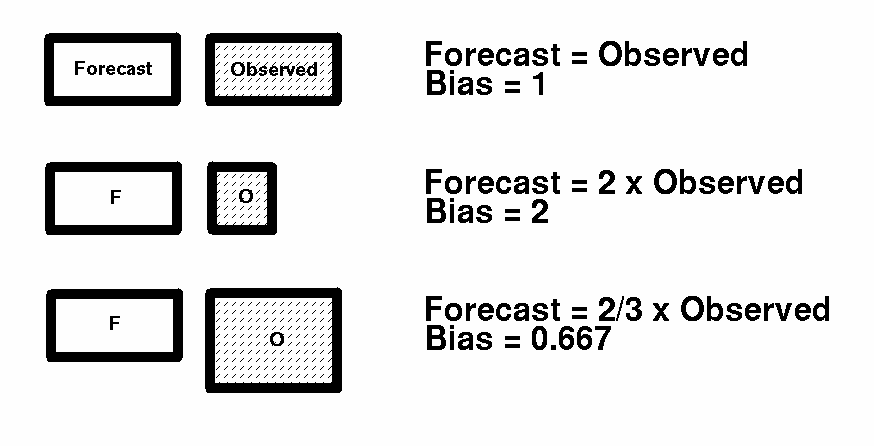Weather Prediction Center
College Park, MD

## WPC Verification -- Threat Score and Bias Computation

 Note: For the computations that follow, the units of "Forecast", "Observed", and "Correct" for a specified precipitation threshold amount are in square kilometers for 24-hourly forecasts and number of stations for 6-hourly forecasts. Threat Score (TS): The formula for computing the TS is Correct / (Forecast + Observed - Correct) For a perfect forecast, Correct = Forecast = Observed to yield a TS of 1. The worst possible forecast, with Correct = 0, yields a TS of zero. Examples:Bias: The basic formula for computing the Bias is Forecast / Observed This quantity gauges the accuracy of areal/station coverage of a specified precipitation threshold amount, regardless of accuracy in location. An ideal forecast would have Forecast = Observed to yield a Bias of 1. Examples:Questions? Comments? Click here to send mail to the WPC.